Problem Solving in the Content Areas

Use the lessons and problems in this series to bridge the gap between basic skills and problem-solving applications.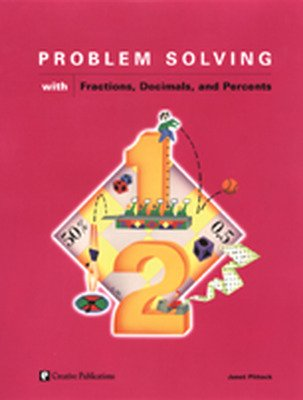Each Problem Solving in the Content Area book contains:

• Problem situations in one math content area to allow your students to practice and apply what they know.
• Sections that begin with a teaching problem that uses the standard 4-step method found in the highly acclaimed series, The Problem Solver.
• Problem sets that include teaching hints, informal assessments, and solutions.

Each book contains 80 pages.

Program Components

•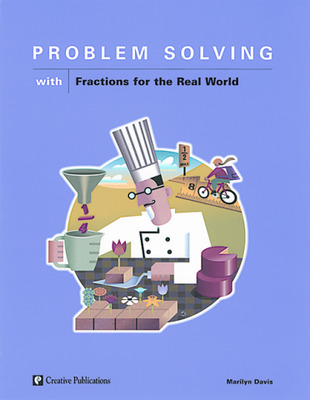Problem Solving with Fractions for the Real World
•Problem Solving with Fractions, Decimals, and Percents
•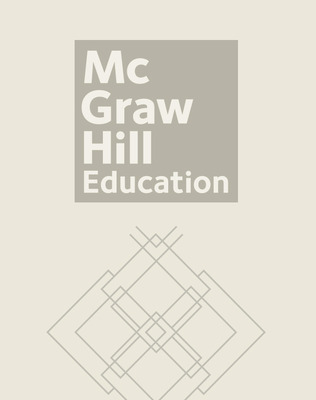Problem Solving with Measurement
•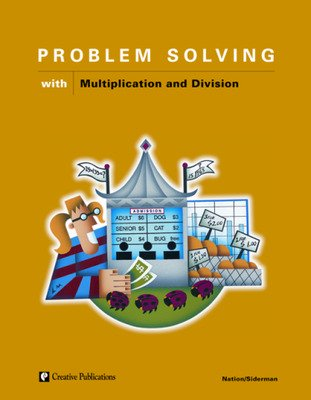Problem Solving with Multiplication and Division
•Problem Solving with Percents in the Real World
•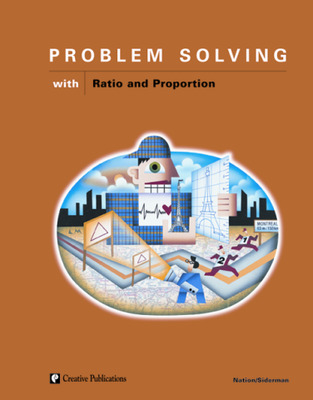Problem Solving with Ratio and Proportion
•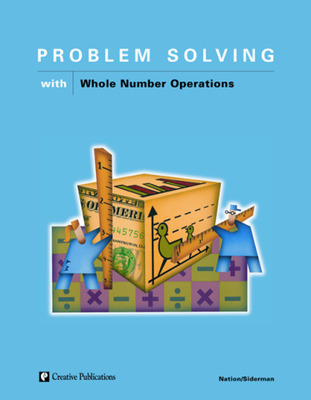Problem Solving with Whole Number Operations
•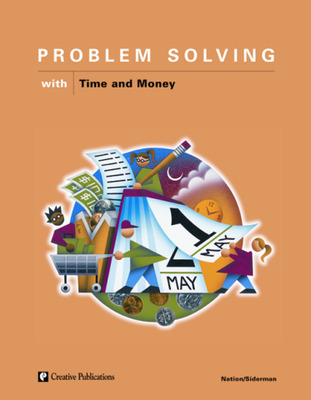Problem Solving with Time and Money
Sort by: